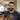# Node Module Caching

March 31, 2017

One of the really powerful features of Node is the ability to naturally separate your code into modules that can be reused throughout your app.

When you require a file in Node, is it instantiated once and that instance passed around where required, or is the module instantiated each time it’s required?

I wasn’t sure so, instead of Googling, I wrote out a quick program to find out.

## Creating visually unique objects

The first thing we need to do is be able to differentiate instances within JavaScript.

``````var a = {}
var b = a
a === b // #=> true

a // #=> {}
a === {} // #=> false

b // #=> {}
b === {} // #=> false``````

This makes sense. We assigned `a` and `b` to the same object when we defined them.

When you call a new object `{}`, it creates a unique instance, but it’s not visually different or immediately understandable.

Ruby `{}.object_id` and Python `id({})` both return the id’s of the object - however JavaScript objects don’t have an id. So in order to see a visual difference with a quick POC, we’ll need some other way.

`Math.random()` generates a unique number which is easy to see.

In the same format as above - replacing the `{}`’s yields a program we can look at and immediately understand without having to think about it.

``````var a = Math.random()
var b = a
a === b // #=> true

a // #=> 0.270583847725012
a === Math.random() // #=> false

b // #=> 0.270583847725012
b === Math.random() // #=> false``````

We can use the above to generate clues about the behaviour of Node.

## Predictions

1. Multiple Instances

IF node creates a new instance of the module every time we require it

THEN we would see `Math.random()` generate a new number every time.

ANY `console.log`’s in the module would appear as many times as the file is required.

2. Single Instance

IF node creates a single instance of the module and passes it into where we require it

THEN we would see `Math.random()` generate only one number.

ANY `console.log`’s in the module would also only appear once.

## Testing

Create the folder and file structure.

``````mkdir node-module-testing
cd node-module-testing

touch index.js instance-1.js instance-2.js random-number.js``````

Write out the testing code.

`random-number.js`

``````var randomNumber = Math.random()

console.log(randomNumber, " => Random Number in Random")

module.exports = randomNumber``````

`instance-1.js`

``````var randomNumber = require("./random-number")

console.log(randomNumber, " => Random Number in Instance 1")

module.exports = randomNumber``````

`instance-2.js`

``````var randomNumber = require("./random-number")

console.log(randomNumber, " => Random Number in Instance 2")

module.exports = randomNumber``````

`index.js`

``````var randomNumber = require("./random-number")
var instance1 = require("./instance-1")
var instance2 = require("./instance-2")

console.log(randomNumber, " => Random Number")
console.log(instance1, " => Instance 1")
console.log(instance2, " => Instance 2")``````

Execute with `node .`

``````0.66966035335478 ' => Random Number in Random'
0.66966035335478 ' => Random Number in Instance 1'
0.66966035335478 ' => Random Number in Instance 2'
0.66966035335478 ' => Random Number'
0.66966035335478 ' => Instance 1'
0.66966035335478 ' => Instance 2'``````

From the results we can clearly see that the instance is created and passed around, this shows that the second prediction is correct.

## Creating Multiple Instances

Let’s create the alternative scenario.

`random-number.js`

``````function randomNumber() {
console.log(randomNumber, " => Random Number in Random")
return Math.random()
}

module.exports = randomNumber``````

`randomNumber` is now a function that is passed around. We will now need to execute it whenever we call it.

We can execute it immediately when we require it - `require('...')()`

`index.js`, `instance-1.js`, and `instance-2.js`;

``var randomNumber = require("./random-number")()``

Execute with `node .`

``````[Function: randomNumber] ' => Random Number in Random'
[Function: randomNumber] ' => Random Number in Random'
0.8149965855422716 ' => Random Number in Instance 1'
[Function: randomNumber] ' => Random Number in Random'
0.7774629927267434 ' => Random Number in Instance 2'
0.8801659041213183 ' => Random Number'
0.8149965855422716 ' => Instance 1'
0.7774629927267434 ' => Instance 2'``````

## Final Notes

You may be thinking we could probably have gotten away with just

``````var randomNumber = Math.random // NOTE: no execution ()'s

console.log(randomNumber, " => Random Number in Random")

module.exports = randomNumber``````

However the above wouldn’t meet all conditions.

Not all code contained within the module is executed. This would only execute the `console.log` once when the module is instantiated, and not every time the module is required.

This may seem minor, however if the `console.log` was replaced with something that was required to run every time, it wouldn’t work as expected and could lead to a fun time debugging.

For example

``````var runOnce = Math.random()

function randomNumber() {
console.log(runOnce, " => Did it change?")
return Math.random()
}

module.exports = randomNumber``````
``````0.6195124356581816 ' => Did it change?'
0.6195124356581816 ' => Did it change?'
...
0.6195124356581816 ' => Did it change?'
...``````

No change. If we encapsulate `runOnce` within the function we see a different result.

``````function randomNumber() {
var runOnce = Math.random()
console.log(runOnce, " => Did it change?")
return Math.random()
}

module.exports = randomNumber``````
``````0.5365654376298239 ' => Did it change?'
0.7857853977341485 ' => Did it change?'
...
0.7507162757727521 ' => Did it change?'
...``````

The above shows the code is executed each time it’s run.Written by Oliver Smit. I love talking about web technologies, good design practices, optimising productivity, and rapid prototyping of projects. Check out my work on Github